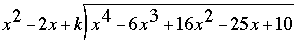SEARCH HOMEMath Central Quandaries & QueriesQuestion from RAM, a parent: If the polynomial x4-6x3+16x2-25x+10 is divided by another polynomial x2-2x+k, the remainder comes out to be x+a. Find k and a. PLEASE GIVE THE METHOD ALSO NOT ONLY THE ANSWER THANKS A LOTHi Ram,

In order to find k & a we must first find the remainder in terms of k. We can find this by using polynomial long division. Perform the divisionThe remainder involves both x and k and you can group the terms involving x to write the remainder

p(k) x + q(k)

where p(k) and q(k) are expressions involving k. But you know the remainder is x + a so

p(k) x + q(k) = x + a

Thus

p(k) = 1 and q(k) = a.

Solve the first equation for k and then substitute the value of k into the second equation and solve for a.

Hope this helps.
Janice and HarleyMath Central is supported by the University of Regina and The Pacific Institute for the Mathematical Sciences.